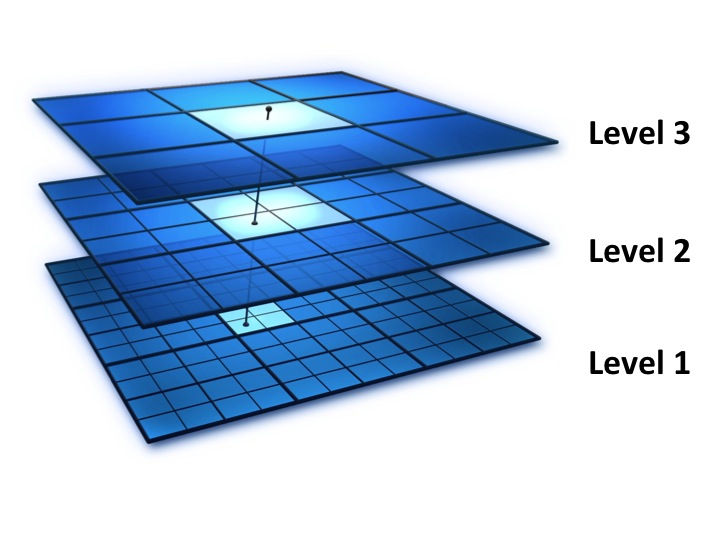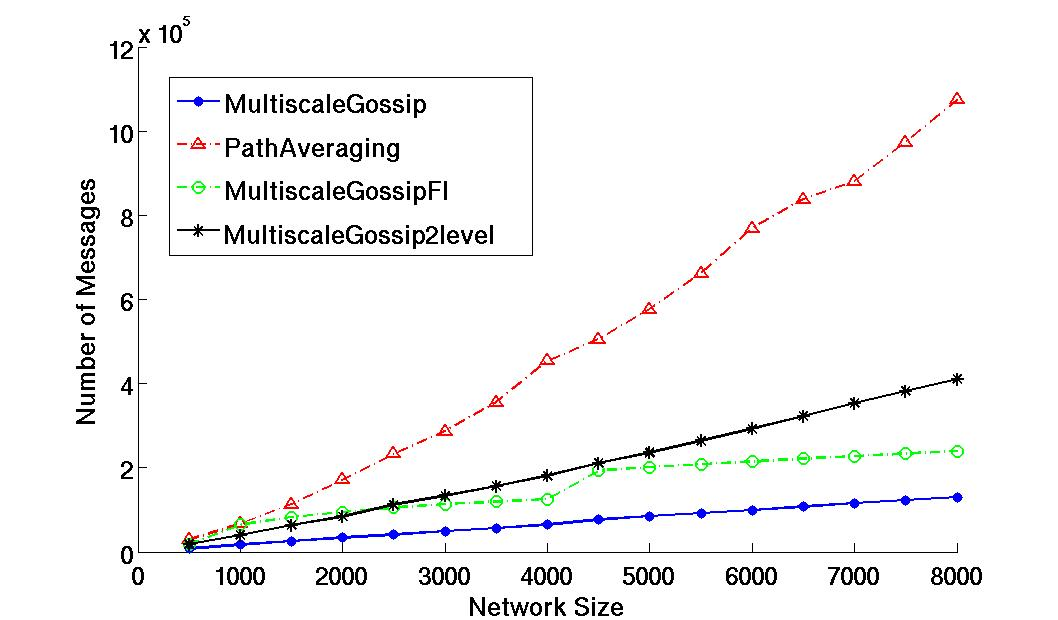## Multiscale Gossip for Fast Averaging

General Description

We consider the problem on distributed averaging in sensor networks. To improve the longevity of a network we need algorithms that can reach consensus to the average using a minimal number of transmissions. In this project we develop an algorithm which converges w.h.p. using O(n loglogn) number of transmissions on Random Geometric Graphs with n nodes. Our algorithm computes the average by hierarchically splitting the graph and solving a number of subproblems whose local averages are then combined. This approach limits the maximum distance that any multi-hop message needs to travel and balances the number of transmissions that each node has to make

1. IEEE Transactions on Signal Processing, arXiv:1011.2235, Submitted.

2. IEEE Distributed Computing in Sensor Systems (DCOSS), vol. 6131, Santa Barbara, Springer Berlin / Heidelberg, pp. 320-333, 06/2010.

Detailed DescriptionWe model a sensor network as a 2D Random Geometric Graph. Each node holds a real value and we are interested in computing the average of the values using only transmissions between neighbouring nodes in the graph. The goal is to minimize the total number of single-hop transmissions.

We logically divide the unit square into cells. At the lowest level we have a lot of small cells while at the top we have few large cells. Assuming each node knows its own coordinates in the unit square and the size of the network n, each node knows which cell it belongs to at each level. At each level only nodes within the same cell are allowed to communicate to compute their cell average. When the computation at one level completes, a representative is selected from each cell to carry the local average estimate one level higher. At that next level only the representatives will participate in the computation. As we move up the levels of our logical hierarchy, the cells get bigger and the local averages are fused into averages over a larger portion of the network until at the top we compute the global average which is then disseminated down to all the nodes. For averaging within each subgraph at any level we can simply use Randomized Gossip.

We achieve very low number of total transmissions by carefully designing our logical hierarchy. Specifically, at the lowest level we have a lot of cells each containing a very small subgraph of the original network. The nodes in the subgraphs are close to each other and communicate directly. At the top level, we have very few large cells but the representative nodes need to use multi-hop communication. We show that by splitting each cell containing q nodes into q^(1-a) cells containing q^a nodes each with a = 2/3 yields linear cost (in number of transmissions) per level. This cost is compute for a level as #cells x #messages per cell x message cost. Where as we move up the hierarchy, the #cells decreases but the message cost increases. With linear (O(n)) total cost per level if we use a slowly increasing number of levels e.g. k=O(loglog(n)), we can get very close to linear cost overall. For more details on the construction and analysis please refer to [1,2].

Evaluation

To evaluate Multiscale Gossip we compare it against Path Averaging, a state of the art gossip algorithm which achieves linear complexity also assuming knowledge of the geographic coordinates of each node and its neighbours in the unit square. As we can see, different variants of Multiscale Gossip converge to the same epsilon=0.0001 accuracy using much less transmissions that Path Averaging. In the figure we see the ideal case for Multiscale Gossip (blue), and implementation with only two levels (black). In both of those cases for each intermediate subgraph we run gossip until convergence and then proceed to the next level. For a practical implementation we run gossip on all subgraphs at the same level for a fixed number of iterations and then proceed (green).Related Publications

1. IEEE Transactions on Signal Processing, arXiv:1011.2235, Submitted. Abstract Download: 1011.2235v1.pdf (948.65 KB)
2. IEEE Distributed Computing in Sensor Systems
(DCOSS), vol. 6131, Santa Barbara, Springer Berlin / Heidelberg, pp. 320-333, 06/2010. Download: dcoss2010.pdf (430.33 KB)